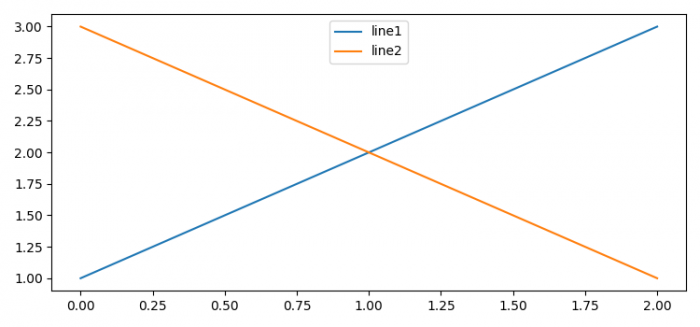# How to label a line in Matplotlib (Python)?

To label a line in matplotlib, we can use label in the argument of plot() method,

## Steps

• Set the figure size and adjust the padding between and around the subplots.
• Plot with label="line1" using plot() method.
• Plot with label="line2" using plot() method.
• To place a legend on the figure, use legend() method.
• To display the figure, use show() method.

## Example

from matplotlib import pyplot as plt
plt.rcParams["figure.figsize"] = [7.50, 3.50]
plt.rcParams["figure.autolayout"] = True
line1, = plt.plot([1, 2, 3], label="line1")
line2, = plt.plot([3, 2, 1], label="line2")
leg = plt.legend(loc='upper center')
plt.show()

## Output